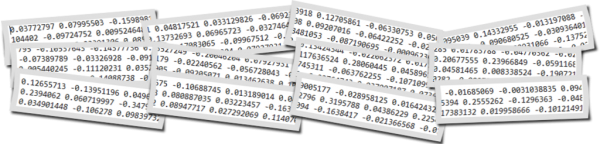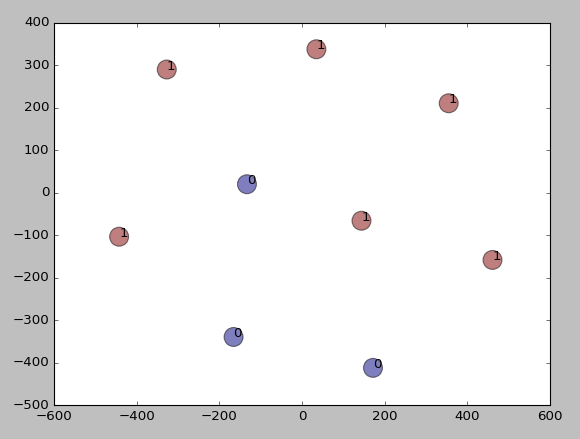# Text Clustering with Word Embedding in Machine LearningText clustering is widely used in many applications such as recommender systems, sentiment analysis, topic selection, user segmentation. Word embeddings (for example word2vec) allow to exploit ordering
of the words and semantics information from the text corpus. In this blog you can find several posts dedicated different word embedding models:

In contrast to last post from the above list, in this post we will discover how to do text clustering with word embeddings at sentence (phrase) level. The sentence could be a few words, phrase or paragraph like tweet. For examples we have 1000 of tweets and want to group in several clusters. So each cluster would contain one or more tweets.

## Data

Our data will be the set of sentences (phrases) containing 2 topics as below:
Note: I highlighted in bold 3 sentences on weather topic, all other sentences have totally different topic.
sentences = [[‘this’, ‘is’, ‘the’, ‘one’,’good’, ‘machine’, ‘learning’, ‘book’],
[‘this’, ‘is’, ‘another’, ‘book’],
[‘one’, ‘more’, ‘book’],
[‘weather’, ‘rain’, ‘snow’],
[‘yesterday’, ‘weather’, ‘snow’],
[‘forecast’, ‘tomorrow’, ‘rain’, ‘snow’],

[‘this’, ‘is’, ‘the’, ‘new’, ‘post’],
[‘this’, ‘is’, ‘about’, ‘more’, ‘machine’, ‘learning’, ‘post’],
[‘and’, ‘this’, ‘is’, ‘the’, ‘one’, ‘last’, ‘post’, ‘book’]]

## Word Embedding Method

For embeddings we will use gensim word2vec model. There is also doc2vec model – but we will use it at next post.
With the need to do text clustering at sentence level there will be one extra step for moving from word level to sentence level. For each sentence from the set of sentences, word embedding of each word is summed and in the end divided by number of words in the sentence. So we are getting average of all word embeddings for each sentence and use them as we would use embeddings at word level – feeding to machine learning clustering algorithm such k-means.

Here is the example of the function that doing this:

```def sent_vectorizer(sent, model):
sent_vec =[]
numw = 0
for w in sent:
try:
if numw == 0:
sent_vec = model[w]
else:
sent_vec = np.add(sent_vec, model[w])
numw+=1
except:
pass

return np.asarray(sent_vec) / numw
```

Now we will use text clustering Kmeans algorithm with word2vec model for embeddings. For kmeans algorithm we will use 2 separate implementations with different libraries NLTK for KMeansClusterer and sklearn for cluster. This was described in previous posts (see the list above).

The code for this article can be found in the end of this post. We use 2 for number of clusters in both k means text clustering algorithms.
Additionally we will plot data using tSNE.

## Output

Below are results

[1, 1, 1, 0, 0, 0, 1, 1, 1]

Cluster id and sentence:
1:[‘this’, ‘is’, ‘the’, ‘one’, ‘good’, ‘machine’, ‘learning’, ‘book’]
1:[‘this’, ‘is’, ‘another’, ‘book’]
1:[‘one’, ‘more’, ‘book’]
0:[‘weather’, ‘rain’, ‘snow’]
0:[‘yesterday’, ‘weather’, ‘snow’]
0:[‘forecast’, ‘tomorrow’, ‘rain’, ‘snow’]

1:[‘this’, ‘is’, ‘the’, ‘new’, ‘post’]
1:[‘this’, ‘is’, ‘about’, ‘more’, ‘machine’, ‘learning’, ‘post’]
1:[‘and’, ‘this’, ‘is’, ‘the’, ‘one’, ‘last’, ‘post’, ‘book’]

Score (Opposite of the value of X on the K-means objective which is Sum of distances of samples to their closest cluster center):
-0.0008175040203510163
Silhouette_score:
0.3498247

Cluster id and sentence:
1 [‘this’, ‘is’, ‘the’, ‘one’, ‘good’, ‘machine’, ‘learning’, ‘book’]
1 [‘this’, ‘is’, ‘another’, ‘book’]
1 [‘one’, ‘more’, ‘book’]
0 [‘weather’, ‘rain’, ‘snow’]
0 [‘yesterday’, ‘weather’, ‘snow’]
0 [‘forecast’, ‘tomorrow’, ‘rain’, ‘snow’]

1 [‘this’, ‘is’, ‘the’, ‘new’, ‘post’]
1 [‘this’, ‘is’, ‘about’, ‘more’, ‘machine’, ‘learning’, ‘post’]
1 [‘and’, ‘this’, ‘is’, ‘the’, ‘one’, ‘last’, ‘post’, ‘book’]Results of text clustering

We see that the data were clustered according to our expectation – different sentences by topic appeared to different clusters. Thus we learned how to do clustering algorithms in data mining or machine learning with word embeddings at sentence level. Here we used kmeans clustering and word2vec embedding model. We created additional function to go from word embeddings to sentence embeddings level. In the next post we will use doc2vec and will not need this function.

Below is full source code python script.

```from gensim.models import Word2Vec

from nltk.cluster import KMeansClusterer
import nltk
import numpy as np

from sklearn import cluster
from sklearn import metrics

# training data

sentences = [['this', 'is', 'the', 'one','good', 'machine', 'learning', 'book'],
['this', 'is',  'another', 'book'],
['one', 'more', 'book'],
['weather', 'rain', 'snow'],
['yesterday', 'weather', 'snow'],
['forecast', 'tomorrow', 'rain', 'snow'],
['this', 'is', 'the', 'new', 'post'],
['this', 'is', 'about', 'more', 'machine', 'learning', 'post'],
['and', 'this', 'is', 'the', 'one', 'last', 'post', 'book']]

model = Word2Vec(sentences, min_count=1)

def sent_vectorizer(sent, model):
sent_vec =[]
numw = 0
for w in sent:
try:
if numw == 0:
sent_vec = model[w]
else:
sent_vec = np.add(sent_vec, model[w])
numw+=1
except:
pass

return np.asarray(sent_vec) / numw

X=[]
for sentence in sentences:
X.append(sent_vectorizer(sentence, model))

print ("========================")
print (X)

# note with some version you would need use this (without wv)
#  model[model.vocab]
print (model[model.wv.vocab])

print (model.similarity('post', 'book'))
print (model.most_similar(positive=['machine'], negative=[], topn=2))

NUM_CLUSTERS=2
kclusterer = KMeansClusterer(NUM_CLUSTERS, distance=nltk.cluster.util.cosine_distance, repeats=25)
assigned_clusters = kclusterer.cluster(X, assign_clusters=True)
print (assigned_clusters)

for index, sentence in enumerate(sentences):
print (str(assigned_clusters[index]) + ":" + str(sentence))

kmeans = cluster.KMeans(n_clusters=NUM_CLUSTERS)
kmeans.fit(X)

labels = kmeans.labels_
centroids = kmeans.cluster_centers_

print ("Cluster id labels for inputted data")
print (labels)
print ("Centroids data")
print (centroids)

print ("Score (Opposite of the value of X on the K-means objective which is Sum of distances of samples to their closest cluster center):")
print (kmeans.score(X))

silhouette_score = metrics.silhouette_score(X, labels, metric='euclidean')

print ("Silhouette_score: ")
print (silhouette_score)

import matplotlib.pyplot as plt

from sklearn.manifold import TSNE

model = TSNE(n_components=2, random_state=0)
np.set_printoptions(suppress=True)

Y=model.fit_transform(X)

plt.scatter(Y[:, 0], Y[:, 1], c=assigned_clusters, s=290,alpha=.5)

for j in range(len(sentences)):
plt.annotate(assigned_clusters[j],xy=(Y[j], Y[j]),xytext=(0,0),textcoords='offset points')
print ("%s %s" % (assigned_clusters[j],  sentences[j]))

plt.show()
```